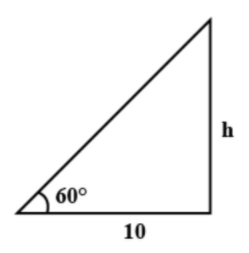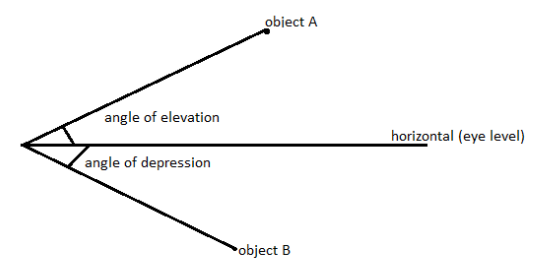QuestionAnswers

# The angle of elevation of the tip of a flagstaff from a point ${\text{10m}}$ due to south of its base is ${\text{6}}{{\text{0}}^{\text{o}}}$. What is the height of the flagstaff correct to the nearest meter?A) ${\text{15m}}$B) ${\text{16m}}$C) ${\text{17m}}$D) ${\text{18m}}$

Hint: In this question, we have been given the angle of elevation of the tip of a flag, and the distance between the base of a flag and the point of elevation angle is also given. So we can apply the formula of ${\text{tan}\theta }$ to find the height of the flag.

Complete step by step solution: Here, we have been given angle of elevation of the tip of a flag is ${\text{6}}{{\text{0}}^{\text{o}}}$ and the distance from a base is${\text{10m}}$ to south.
So, using the above information first we draw the following diagramAs we know ${{tan\theta = }}\dfrac{{{\text{opposite side}}}}{{{\text{adjacent side}}}}$
So, form the figure
We have,
${\text{tan6}}{{\text{0}}^{\text{o}}}{\text{ = }}\dfrac{{\text{h}}}{{{\text{10}}}}$
As we know ${\text{tan6}}{{\text{0}}^{\text{o}}}{\text{ = }}\sqrt {\text{3}}$, substitute this value in the above expression
$\Rightarrow \sqrt {\text{3}} {\text{ = }}\dfrac{{\text{h}}}{{{\text{10}}}}$
$\Rightarrow {\text{h = 10} \times }\sqrt {\text{3}}$
As we know $\sqrt 3 = 1.732$, substituting this value, we get,
$\Rightarrow {\text{h} = 10}\times{1.732}$
$\Rightarrow {\text{h = 17}}{\text{.32}}$
$\Rightarrow {\text{h}} \approx {\text{17}}$m
Thus, the height of the flagstaff correct to the nearest meter is ${\text{17m}}$.

Hence, option C. ${\text{17m}}$ is the correct answer.

Note: When we look at an object above our eye level, our line of sight will make an angle with the horizontal eye level. This angle is called the angle of elevation. If we are at the top of some building or a tower and we look at some object below our eye level. Then the angle that the line of sight makes with the horizontal eye level is called the angle of depression.We use the concept of trigonometry to solve this problem. In practical day to day life, we use these concepts of the angle of elevation and trigonometry to find the height of buildings, towers etc.